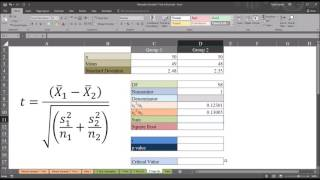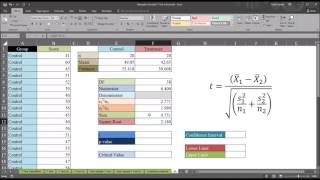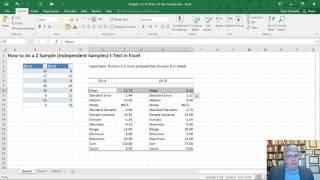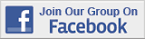Excel Tips & Tutorials:
Hypothesis Tests with One and Two Samples
 Suggest a Link Alphabetize Page Printer-Friendly List
It Has to Start Somewhere
Watch and Learn
Watch and Learn
Watch and Learn
Watch and Learn
 Independent Samples T-Test from Only Sample Size, Mean, and Standard DeviationTodd Grande shows how to conduct t-test from descriptive statistics (7:38)How to Create Confidence Intervals for Difference of ProportionsDavid Houston works through political examples (20:43).Calculating the 95% Confidence Interval after a T-TestFrom Todd Grande (6:57)How to do a Two Sample (Independent) t-Test in ExcelFrom David Dunaetz (8:45)Independent Samples T-Test from Only Sample Size, Mean, and Standard DeviationTodd Grande shows how to conduct t-test from descriptive statistics (7:38)How to Create Confidence Intervals for Difference of ProportionsDavid Houston works through political examples (20:43).Calculating the 95% Confidence Interval after a T-TestFrom Todd Grande (6:57)Independent Samples T-Test from Only Sample Size, Mean, and Standard DeviationTodd Grande shows how to conduct t-test from descriptive statistics (7:38)How to Create Confidence Intervals for Difference of ProportionsDavid Houston works through political examples (20:43).Independent Samples T-Test from Only Sample Size, Mean, and Standard DeviationTodd Grande shows how to conduct t-test from descriptive statistics (7:38)Over 18,000 Members!